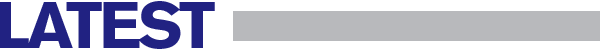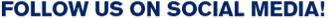Science

# Pi smashing world record

By Sam Tilley

The concept of pi has enthralled scientists, mathematicians, and astronomers since it was first uncovered. Whilst the first attempts at unraveling the mystery of circles have been dated back to Ancient Egyptian times, Google employee Emma Haruka Iwao has calculated the figure to 31.4 trillion digits; largely thanks to the Google Cloud computing service. The previous world record, a mere 22 trillion digits, was calculated by Peter Trueb in 2016.

The value of pi is calculated by taking the circumference of any given circle and dividing it by its diameter. Pi is used across the word in fields as diverse as space exploration and supercomputing as the value can be taken to represent any one of waves, cylinders, and circles.

The record, certified by Guinness World Records, was calculated thanks to the program ‘y-cruncher’; one of a growing number of supercomputers that have been custom-made to calculate as many digits as possible. The Chudnovky algorithm, most used algorithm for calculating pi, is so complex that it becomes increasingly expensive to calculate more and more digits.

In addition to this, the longer the calculations take, the more prone the computer is to crashes or hardware failure. Iwao has already stated that she hopes to break her own record and stated, quite correctly, that “there is no end to pi”.

#### Latest